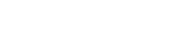# math.sin

`FLOATmath.sinFLOATx`

Available inall subroutines.

Computes the sine of its argument `x`, measured in radians.

## Parameters

`x` - Floating point value representing an angle in radians.

## Return Value

Upon successful completion, this function returns the sine of `x`.

If `x` is `math.NAN`, a NaN will be returned.

If `x` is ±0, `x` will be returned.

If `x` is `math.POS_INFINITY` or `math.NEG_INFINITY`, a domain error occurs and a NaN will be returned.

If `x` is subnormal, a range error occurs and `x` will be returned.

## Errors

• If the `x` argument is `math.POS_INFINITY` or `math.NEG_INFINITY`, then `fastly.error` will be set to `EDOM`.
• If the `x` argument is subnormal, then `fastly.error` will be set to `ERANGE`.

## Example

``````declare local var.fi FLOAT;declare local var.fo FLOAT;
set var.fi = math.PI;set var.fi /= 6;set var.fo = math.sin(var.fi);``````

BETA